# Use properties of limits and algebraic methods to find the limit, if it exists. (If the limit is infinite, enter '∞' or '-∞', as appropriate. If the limit does not otherwise exist, enter DNE.)

Use properties of limits and algebraic methods to find the limit, if it exists. (If the limit is infinite, enter '' or '-', as appropriate. If the limit does not otherwise exist, enter DNE.)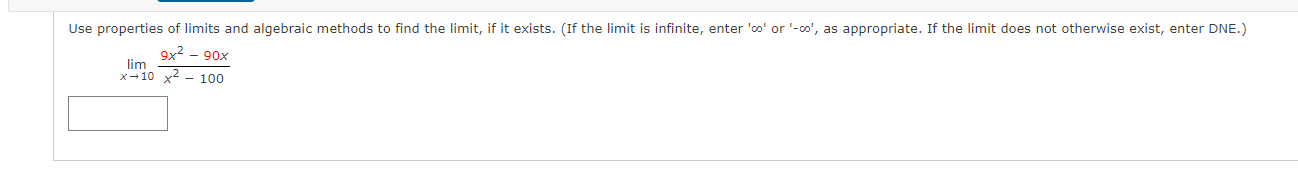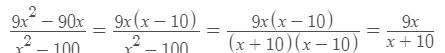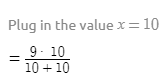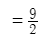#### Earn Coin

Coins can be redeemed for fabulous gifts.

Similar Homework Help Questions
• ### Use properties of limits and algebraic methods to find the limit, if it exists. (If the limit is infinite, enter '∞' or '-∞', as appropriate. If the limit does not otherwise exist, enter DNE.)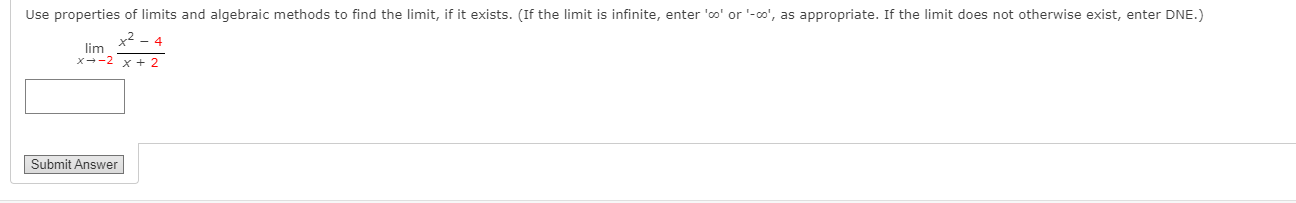Use properties of limits and algebraic methods to find the limit, if it exists. (If the limit is infinite, enter '∞' or '-∞', as appropriate. If the limit does not otherwise exist, enter DNE.)

• ### Use properties of limits and algebraic methods to find the limit, if it exists. (If the limit is infinite, enter '∞' or '-∞', as appropriate. If the limit does not otherwise exist, enter DNE.)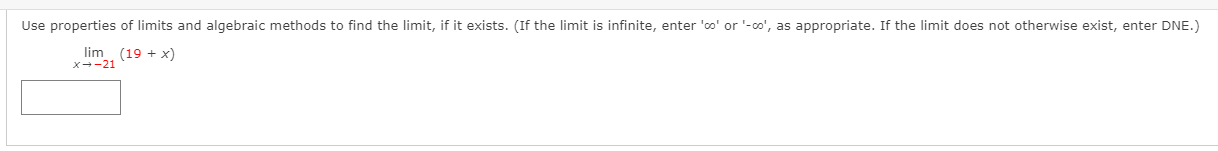Use properties of limits and algebraic methods to find the limit, if it exists. (If the limit is infinite, enter '∞' or '-∞', as appropriate. If the limit does not otherwise exist, enter DNE.)

• ### Use properties of limits and algebraic methods to find the limit, if it exists. (If the limit is infinite, enter '∞' or '-∞', as appropriate. If the limit does not otherwise exist, enter DNE.)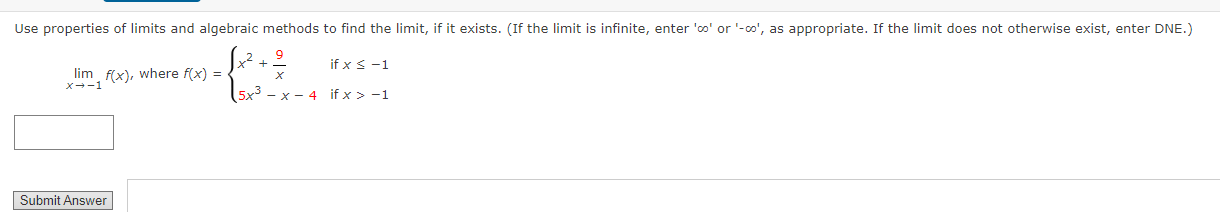Use properties of limits and algebraic methods to find the limit, if it exists. (If the limit is infinite, enter '∞' or '-∞', as appropriate. If the limit does not otherwise exist, enter DNE.)

• ### Use properties of limits and algebraic methods to find the limit, if it exists. (If the limit is infinite, enter '∞' or '-∞', as appropriate. If the limit does not otherwise exist, enter DNE.)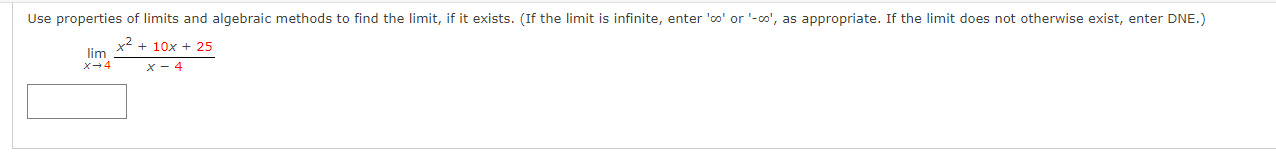Use properties of limits and algebraic methods to find the limit, if it exists. (If the limit is infinite, enter '∞' or '-∞', as appropriate. If the limit does not otherwise exist, enter DNE.)

• ### Use properties of limits and algebraic methods to find the limit, if it exists. (If the limit is infinite, enter '∞' or '-∞', as appropriate. If the limit does not otherwise exist, enter DNE.)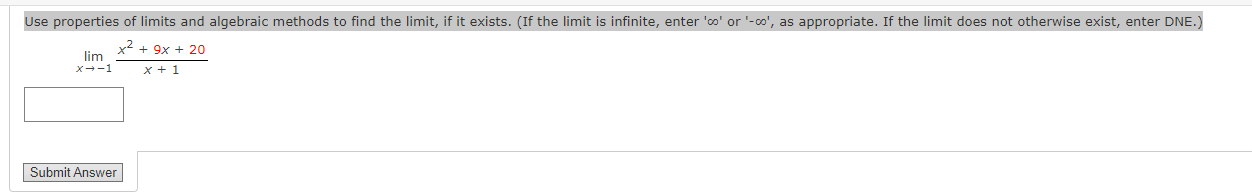Use properties of limits and algebraic methods to find the limit, if it exists. (If the limit is infinite, enter '∞' or '-∞', as appropriate. If the limit does not otherwise exist, enter DNE.)$$\lim _{x \rightarrow-1} \frac{x^{2}+9 x+20}{x+1}$$

• ### Use properties of limits and algebraic methods to find the limit, if it exists. (If the...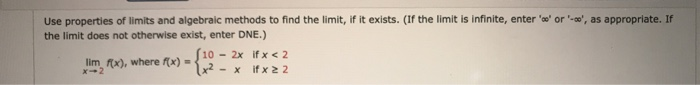Use properties of limits and algebraic methods to find the limit, if it exists. (If the limit is infinite, enter 'se' or '-co', as appropriate. If the limit does not otherwise exist, enter DNE.) 10 - 2x if x < 2 lim Rx), where f(x) = - X if x 22

• ### Complete the table and predict the limit, if it exists. (Round your answers to three decimal places. If the limit is infinite, enter '∞' or '-∞', as appropriate. If the limit does not otherwise exist, enter DNE.)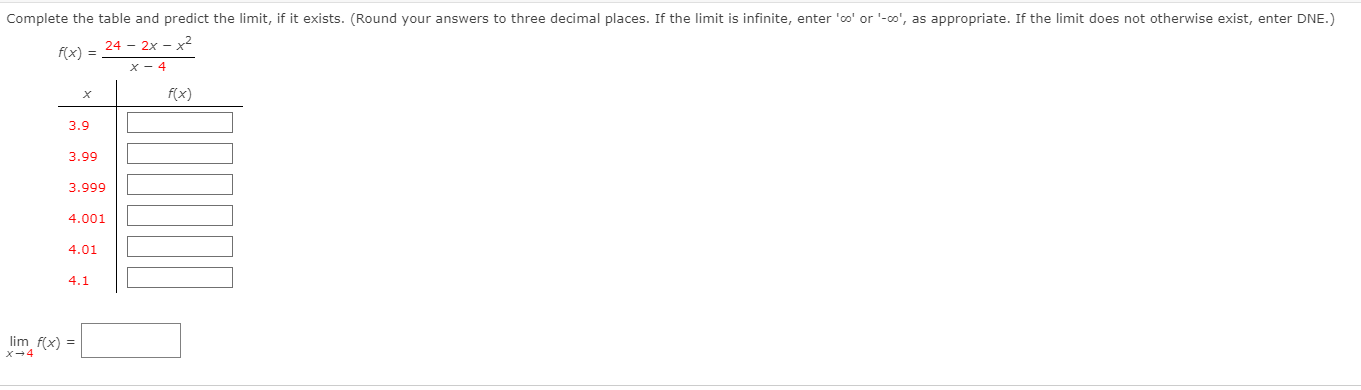Complete the table and predict the limit, if it exists. (Round your answers to three decimal places. If the limit is infinite, enter '∞' or '-∞', as appropriate. If the limit does not otherwise exist, enter DNE.)

• ### DETAILS HARMATHAPBR1 9.1.009. Use properties of limits and algebraic methods to find the limit, if it...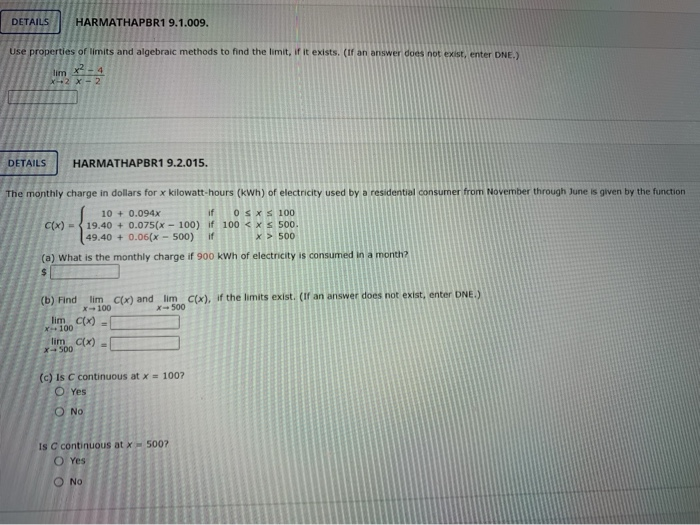DETAILS HARMATHAPBR1 9.1.009. Use properties of limits and algebraic methods to find the limit, if it exists. (If an answer does not exist, enter ONE.) lim XX-2 DETAILS HARMATHAPBR1 9.2.015. The monthly charge in dollars for x kilowatt-hours (kWh) of electricity used by a residential consumer from November through June is given by the function 10 + 0.094x if O SXS 100 C(x) - 19.40 + 0.075(x - 100) if 100 < x < 500. 49.40 + 0.06(x - 500)...

• ### Evaluate the limit, if it exists. (If an answer does not exist, enter DNE.) lim V...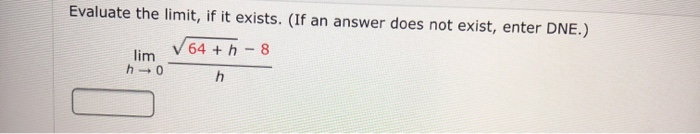Evaluate the limit, if it exists. (If an answer does not exist, enter DNE.) lim V 64 + - 8 h0

• ### Use the graph to determine the limit. (If an answer does not exist, enter DNE.)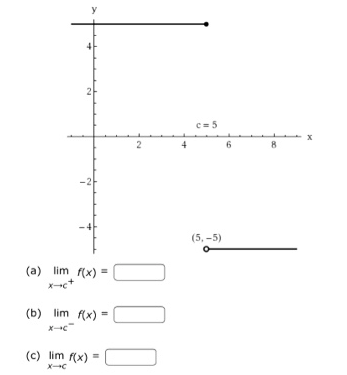Use the graph to determine the limit. (If an answer does not exist, enter DNE.) Is the function continuous at x=5 ?YesNo